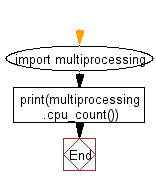﻿ Python: Find out the number of CPUs using - w3resource# Python: Find out the number of CPUs using

## Python Basic: Exercise-47 with Solution

Write a Python program to find out the number of CPUs using.

Sample Solution:-

Python Code:

``````import multiprocessing
print(multiprocessing.cpu_count())
```
```

Sample Output:

```4
```

Flowchart:Python Code Editor:

Have another way to solve this solution? Contribute your code (and comments) through Disqus.

What is the difficulty level of this exercise?

Test your Python skills with w3resource's quiz

﻿

## Python: Tips of the Day

List comprehension:

```>>> m = [x ** 2 for x in range(5)]
>>> m
[0, 1, 4, 9, 16]
```